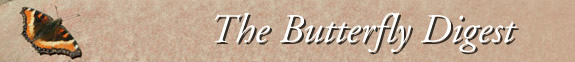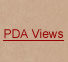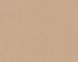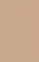Jump to : Today April 1, 2020 March 31, 2020 March 30, 2020 March 29, 2020 March 28, 2020 March 27, 2020 March 26, 2020 March 25, 2020 March 24, 2020 March 23, 2020 March 22, 2020 March 21, 2020 March 20, 2020 March 19, 2020 March 18, 2020 March 17, 2020 March 16, 2020 March 15, 2020 March 14, 2020 March 13, 2020 March 12, 2020 March 11, 2020 March 10, 2020 March 9, 2020 March 8, 2020 March 7, 2020 March 6, 2020 March 5, 2020 March 4, 2020 March 3, 2020     |    View All Lists    |    FAQ
 List: western_monarchs Date: Wed, 25 Mar 2020 20:22:33 -0700 From: 'Dave Wikle' [western_monarchs] Subject: =?UTF-8?Q?RE:_=5Bwestern=5Fmonarchs=5D_What=E2=80=99s_Ha?= =?UTF-8?Q?ppening_to_the_=28Western=29_Monarch?= =?UTF-8?Q?_Butterfly_Population=3F_=28New_York?= =?UTF-8?Q?_Times=2C_March_20/20=29?= ------=_NextPart_000_0013_01D602E3.1E769D10Content-Type: text/plain; charset="UTF-8"Content-Transfer-Encoding: quoted-printableThis sounds an awful lot like quantum flux, Heisenberg uncertainty and the =observer effect. If you look for it, it isn=E2=80=99t what you were lookin=g for anymore. Just by looking for it skews the data. According to Susski=nd, entropy is now information, not just randomness and chaos. Now look wh=at you=E2=80=99ve done.=20From: [mailto:western_monarchs@yahoogroups=..com]=20Sent: Wednesday, March 25, 2020 8:10 AMTo: Francis Xavier Villablanca [western_m=onarchs]Subject: Re: [western_monarchs] What=E2=80=99s Happening to the (Western) M=onarch Butterfly Population? (New York Times, March 20/20)=20=20=20Whether the distribution of tagged butterflies moving away from overwinteri=ng sites in the West is (was) random or preferential depending on=20site of origin can=E2=80=99t be determined from this data set. There are to=o many unknowns. The number one issue is the distribution and density of th=e human population along with their inclination to report sightings/recaptu=res.=20Recaptures and sightings are human density centric. That=E2=80=99s very app=arent in the JN first sightings data. When analyzed by latitude, the JN sig=htings spike with each major city from SA to KC in the midwest. Further, th=ere are gaps each year in rural areas at latitudes where human density is l=ow and the inclination to report sightings is also low. And then there is w=ind direction and wind speed that varies with the seasons and latitudes. Mo=narchs like a tail wind and that has to factor in to the interpretation. Th=ere are also issues of numbers tagged per site and dates of departure which= are likely to be later for cooler overwintering sites further up the coast=... Monarchs seek out more humid sites as well and there are probably other =things we don=E2=80=99t know. In my view, we are left with two reasonable h=ypotheses both of which require further testing.=20This scenario is a bit like tracking the impact of the movements of those w=ho are shedding the Covid-19 virus. The impact of those moving into low den=sity populations may be relatively low and delayed while those moving into =high density close contact populations would be much greater and more easil=y detected - and more serious overall since encounters with or spread to th=ose who are most like to be seriously affected would increase.=20Be safe out there.=20------=_NextPart_000_0013_01D602E3.1E769D10Content-Type: text/html; charset=UTF-8Content-Transfer-Encoding: quoted-printable

This sounds an awful =lot like quantum flux, Heisenberg uncertainty and the observer effect. =C2==A0If you look for it, it isn=E2=80=99t what you were looking for anymore.==C2=A0 Just by looking for it skews the data.=C2=A0 According to Susskind, =entropy is now information, not just randomness and chaos.=C2=A0 Now look w=hat you=E2=80=99ve done.

<=o:p>

From: western_m= [mailto:]
Se=nt: Wednesday, March 25, 2020 8:10 AM
To: Francis Xavier Vill=ablanca [western_monarchs]
Subject:= Re: [western_monarchs] What=E2=80=99s Happening to the (Western) Monar=ch Butterfly Population? (New York Times, March 20/20)

Whether the distribution of tagge=d butterflies moving away from overwintering sites in the West is (was) ran=dom or preferential depending on
site of origin can=E2=80=99t be determ=ined from this data set. There are too many unknowns. The number one issue =is the distribution and density of the human population along with their in=clination to report sightings/recaptures.
Recaptures and sightings are =human density centric. That=E2=80=99s very apparent in the JN first sightin=gs data. When analyzed by latitude, the JN sightings spike with each major =city from SA to KC in the midwest. Further, there are gaps each year in rur=al areas at latitudes where human density is low and the inclination to rep=ort sightings is also low. And then there is wind direction and wind speed =that varies with the seasons and latitudes. Monarchs like a tail wind and t=hat has to factor in to the interpretation. There are also issues of number=s tagged per site and dates of departure which are likely to be later for c=ooler overwintering sites further up the coast.. Monarchs seek out more hum=id sites as well and there are probably other things we don=E2=80=99t know.= In my view, we are left with two reasonable hypotheses both of which requi=re further testing.

This scenario is a bit like tracking the impact= of the movements of those who are shedding the Covid-19 virus. The impact =of those moving into low density populations may be relatively low and dela=yed while those moving into high density close contact populations would be= much greater and more easily detected - and more serious overall since enc=ounters with or spread to those who are most like to be seriously affected =would increase.

Be safe out there.

__._,_.___
=20=20=20=20=20=20=20=20=20=20=20=20

Posted by: "Dave Wikle" <> =
=
=20=20=20=20
=20=20=20=20=20=20=20=20=20 =20=20=20
__,_._,___
------=_NextPart_000_0013_01D602E3.1E769D10--